#### You may also likeMake a set of numbers that use all the digits from 1 to 9, once and once only. Add them up. The result is divisible by 9. Add each of the digits in the new number. What is their sum? Now try some other possibilities for yourself!### Have You Got It?

Can you explain the strategy for winning this game with any target?### Counting Factors

Is there an efficient way to work out how many factors a large number has?

# American Billions

##### Age 11 to 14Challenge Level

We have received solutions from Emily and Ellie from Fitzharrys School, Victor from St. Paul's in Brazil, Jack from Springwood Academy, Rebecca from Loughborough High School, Komal from Alexandra School in India, Sam, Nathan and Dan from Netherthong Primary School, Anurag from Queen Elizabeth's Grammar School in Horncastle, Tom, Jake, Harry and Michael from Corsham Primary School, George from Onslow St. Audrey's School, Ali from Riccarton High School in Christchurch, New Zealand, and Luke from Cottenham in Cambridgeshire.

Victor found that 12365 could be made a little longer:

Yes, 123654 is divisible by 6, but they can't go any futher than that.

He had a go at  finding the longest number you can make that satisfies the rules of the game. He managed a seven digit number:

7836542 is my attempt, I didn't manage to use all of the digits.

Jack found a six digit number that satisfied the rules:

147258

Emily & Ellie found a seven digit number:

9632581

It is possible to find a ten digit number that satisfies the rules. It is:

3816547290

since

3 is divisible by 1
38 is divisible by 2
381 is divisible by 3
3816 is divisible by 4
38165 is divisible by 5
381654 is divisible by 6
3816547 is divisible by 7
38165472 is divisible by 8
381654729 is divisible by 9
3816547290 is divisible by 10

Tom, Jake, Harry and Michael worked it out like this:

We realised that the 0 had to go at the end (Units) for it to be a multiple of 10.
We then knew the 5th number would have to be a 5 - because it's the only other multiple of 5.
We realised that any number divisible by 2 , 4, 6 or 8 had to be an even number.
From this we decided the billion digit must be odd and the following digits even and so on:

Odd Even Odd Even 5 Even Odd Even Odd 0

We used the elimination method starting with 98 and went down to 92 and kept on going down. Eventually we found the answer: 3816547290.

Ali worked it out like this:

Let A B C D E F G H I J represent the 10 digits.

The number itself is divisible by 10, so J must be 0.

Since the number formed by the first 5 digits from the left is divisible by 5, E must be 5 or 0, but since we have used 0 already, E must be 5.

Since AB, ABCD, ABCDEF and ABCDEFGH must be divisible by 2, 4, 6 and 8 respectively, they must all be even. Therefore B, D, F and H must be even numbers.

That leaves 1, 3, 7 and 9 for A, C, G and I.

Ali then started listing the possible combinations, and eliminating those that did not fit:

AB could be any of the following: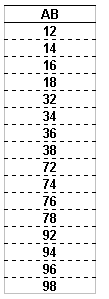The number formed by the first 3 digits from the left is divisible by 3, so the digits must add up to a number that is divisible by 3.

The possible values of ABC can now be identified: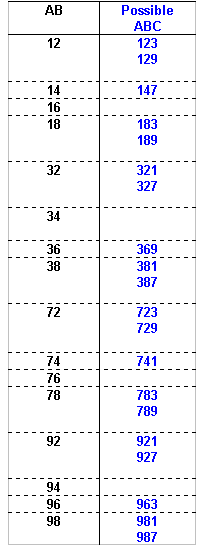The number formed by the first 4 digits from the left is divisible by 4, so the number formed by the last two digits of the number must also be divisible by 4 (since all multiples of 100 are divisible by 4 we can ignore the digits in the hundreds and thousands column).

The possible values of ABCD can now be identified: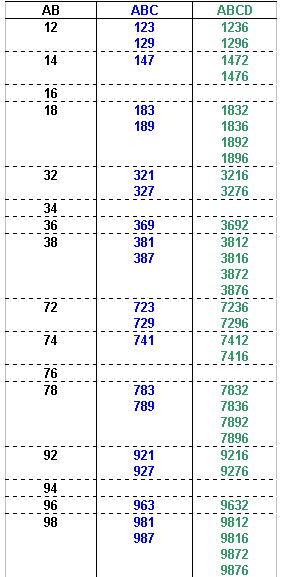We know that the fifth digit must be 5, so the possible values of ABCDE can now be identified: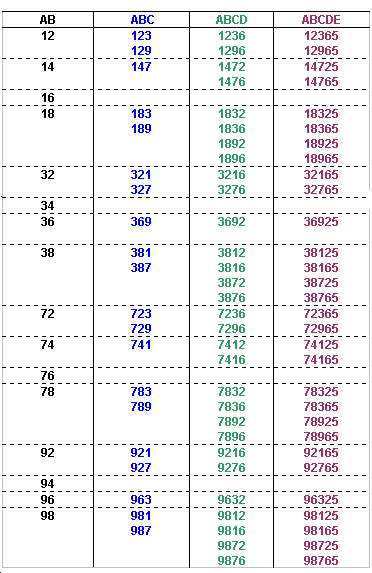The number formed by the first 6 digits from the left is divisible by 6, so it must be even, and the digits must add up to a number that is divisible by 3 (since it must be a multiple of 3).

The possible values of ABCDEF can now be identified: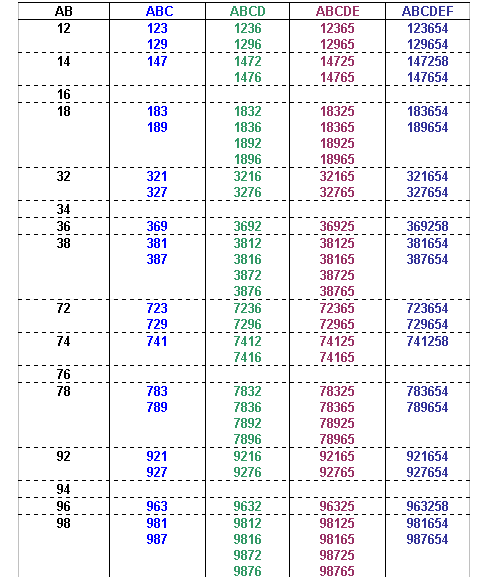The number formed by the first 7 digits from the left is divisible by 7, and the seventh digit must be odd.

The possible values of ABCDEFG can now be identified: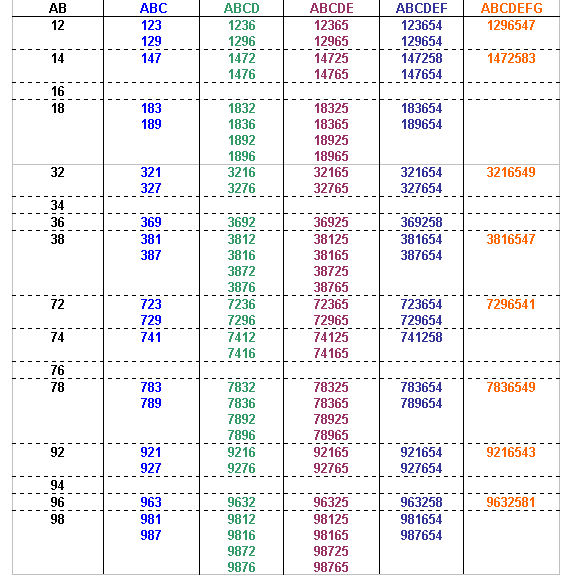The number formed by the first 8 digits from the left is divisible by 8, so the number formed by the last three digits of the number must also be divisible by 8 (since all multiples of 1000 are divisible by 8 we can ignore the digits in the thousands, ten thousands and hundred thousands column).

That leaves only one option for ABCDEFGH: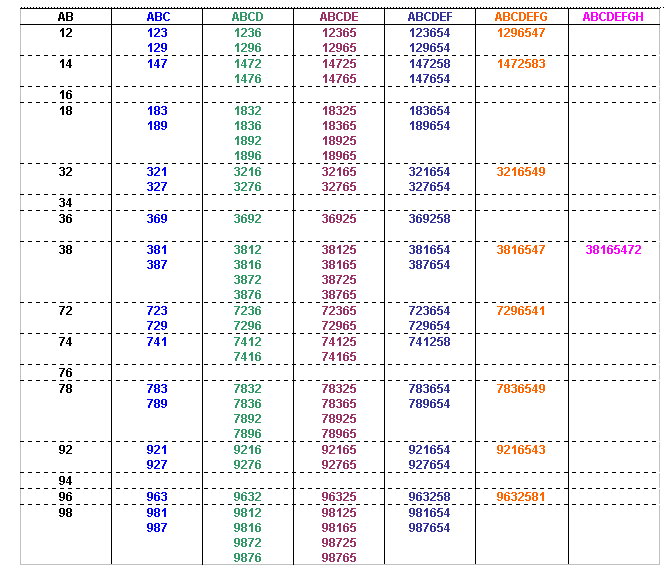Therefore ABCDEFGHIJ can only be:
3816547290

Here is Anurag's solution.

Luke explained his reasoning like this:

I knew that the last digit was zero because only numbers that have zero at the end are divisible by ten.

xxxxxxxxx0

Numbers that are divisible by 5 end in 5 or 0, and 0 has already been used so the fifth digit must be 5.

xxxx5xxxx0

Since an odd number is not divisible by an even number, the pattern must go odd, even, odd, even,..

The second digit is even and, since multiples of 100 are divisible by 4, the number formed by the first 4 digits from the left will be divisible by 4 if the number formed by the last two digits is divisible by 4,

so, ignoring the first two digits,
we know that the fourth digit has to be 2 or 6 since 14, 34, 74, 94, 18, 38, 78 and 98 are not divisible by 4. (The third digit must be odd, and not 5).

So these are our options:

xxx25xxxx0

xxx65xxxx0

The sixth digit is even and, since multiples of 200 are divisible by 8, the number formed by the first 8 digits from the left will be divisible by 8 if the number formed by the last two digits is divisible by 8,

so, ignoring the first six digits
we know that the eighth digit has to be 2 or 6 since 14, 34, 74, 94, 18, 38, 78 and 98 are not divisible by 8. (The seventh number must be odd, and not 5).

Since the fourth digit must be 2 or a 6 as well that means that the second and sixth digits must be 4 or 8.

So these are our options:

x4x258x6x0

x4x658x2x0

x8x254x6x0

x8x654x2x0

This is the bit my Dad helped with. The sum of the first 3 digits must be divisible by 3. Also the sum of the first 6 digits must be divisible by 3. Therefore the sum of the fourth, fifth and sixth digits must be divisible by 3.

So these are our options now:

x4x258x6x0

x8x654x2x0

The first 3 digits must add up to 3.

This gives us the following options:

147258x6x0

741258x6x0

183654x2x0

381654x2x0

189654x2x0

981654x2x0

789654x2x0

987654x2x0

Because the sixth digit is even, and the eighth digit is either 2 or 6,
we can can only have 16, 32, 72, or 96 for the seventh and eighth digits (they are divisible by 8),
and these numbers can't have been used elsewhere.

The ninth digit can be anything because the sum of all the digits 1 to 9 is divisible by 9.

This gives us the following options:

1472589630

7412589630

1836547290

3816547290

1896543270

1896547230

9816543270

9816547230

7896543210

9876543210

Finally, if we check that the numbers formed by the first 7 digits from the left are divisible by 7, we find that the only possible answer is

3816547290

Rebecca also found 3816547290. She sent us this explanation:

We can immediately say that the last digit has to be 0 because the whole number has to be divisible by 10.

The 5th digit:

The 0 has already been used, excluding the chances of the 5th digit being anything other than 5, as the 5th digit has to be divisible by 5.

Even and Odd:

We can also figure out that the 2nd, 4th, 6th, and 8th digits have to be even because no multiple of 2, 4, 6 or 8 can be odd. This means that the 1st, 3rd, 5th, 7th and 9th digits must be odd!

The 4th and 8th digits:

Any hundred can be divided by 4 so we have to focus on the 3rd and 4th digits. In the 4 times table, after every odd ten comes a 2 or a 6. For example: 32, 76, 52, 96 etc?
We therefore know that digit 4 can only be 2 or 6, and because 8 is double 4, this rule applies to the 8th digit too.
This also means that the 2nd and 6th digits can only be 4 or 8.

What next?

After this, the quickest method is to use trial and error, in a structured manner, starting at either the smallest or largest possible number.

Komal sent us this explanation, which demonstrates what a structured approach might look like:

Let our number be -

A B C D E F G H I J

Since our 10-digit number should be divisible by 10 hence the digit J should be 0.
Also our 5-digit number should be divisible by 5. Hence E should be 0/5.
Each number comes only once and J is 0 therefore E will be 5.

The number becomes -

A B C D 5 F G H I 0

Now, our 2-digit number should be divisible by 2 therefore B will be an even number. Similarly 4-digit, 6-digit and 8-digit numbers will also be even numbers.
Therefore B, D, F and H are even numbers i.e. 2, 4, 6, 8.
This implies that A, C, G and I can be the remaining options i.e. 1, 3, 7, 9

For digit B, we know that ABCD is a multiple of 4. The multiples of 4 are 4, 8, 12, 16, 20, 24, 28, 32, 36, 40... Since C is an odd number (1, 3, 7, or 9) and D is an even number, the number D cannot be 4, 8, 0 because these numbers require the previous number to be an even number for the number ABCD to be divisible by 4. Therefore D can only be 2 or 6.

Similarly the 8-digit number is divisible by 8 and hence should be divisible by 4. Using the divisibility rule for 4 we can conclude that H can only be 2 or 6.

This implies that B and F can only be 4 or 8 (the remaining two numbers).

The number of options for the final number are -
A - 1/3/7/9
B - 4/8
C - 1/3/7/9
D - 2/6
E - 5
F - 4/8
G - 1/3/7/9
H - 2/6
I - 1/3/7/9
J - 0

For a number to be divisible by 3, the sum of its digits should be a multiple of 3. Now ABC and ABCDEF are both multiples of 3.
If B is 4 then the sum of A and C can be 2, 5, 8, 11, 14, 17, ... Therefore A and C can be (1,7), (7,1)
If B is 8 then the sum of A and C can be 1, 4, 7, 10, 13, 16, ... Therefore
A and C can be (1,3), (3,1), (1,9), (9,1), (3,7), (7,3)

Option 1: ABC -> 147
F is 4/8 hence F will be 8. E is 5. D will be 2 because 147258 will be a
multiple of 6.
1472583 is a multiple of 7 but 14725836 is not a multiple of 8.
1472589 is not a multiple of 7.

Option 2: ABC -> 741
F is 4/8 hence F will be 8. E is 5. D will be 2 because 741258 will be a
multiple of 6.
7412583 is not a multiple of 7.
7412589 is not a multiple of 7.

Option 3: ABC -> 183
F is 4/8 hence F will be 4. E is 5. D will be 6 because 183654 will be a
multiple of 6.
1836547 is not a multiple of 7.
1836549 is not a multiple of 7.

Option 4: ABC -> 381
F is 4/8 hence F will be 4. E is 5. D will be 6 because 381654 will be a
multiple of 6.
3816547 is a multiple of 7 and 38165472 is a multiple of 8 and 381654729 is
a multiple of 9 and 3816547290
is a multiple of 10.
3816549 is not a multiple of 7.

Option 5: ABC -> 189
F is 4/8 hence F will be 4. E is 5. D will be 6 because 189654 will be a
multiple of 6.
1896543 is not a multiple of 7.
1896547 is not a multiple of 7.

Option 6: ABC -> 981
F is 4/8 hence F will be 4. E is 5. D will be 6 because 981654 will be a
multiple of 6.
9816543 is not a multiple of 7.
9816547 is not a multiple of 7.

Option 7: ABC -> 387
F is 4/8 hence F will be 4. E is 5. D will be 6 because 387654 will be a
multiple of 6.
3876541 is not a multiple of 7.
3876549 is not a multiple of 7.

Option 8: ABC -> 783
F is 4/8 hence F will be 4. E is 5. D will be 6 because 783654 will be a
multiple of 6.
7836541 is not a multiple of 7.
7836549 is not a multiple of 7.

Therefore the longest number that satisfies the given condition is
3816547290.

Sam, Nathan and Dan used the rules for divisibility they knew:

The first rule did not help us so we ignored it.
We knew that rule 10 would be 0 and rule 5 would be 5.
Rule 2 would be even.
To figure out rule 3 add all the digits together and if the digital root is 3,6 or 9 it is divisible by 3.
Rule 4 must be even and the last 2 has to be divisible by 4.
Rule 6 add all the units together and if it is divisible by 3 and the full number is divisble 2 it is right.
Rule 7 use one of the odd numbers that is left.
Rule 8 has to be even and the last 3 digits must add up to be divisible by 8.
Rule 9 just use the last digit that is left or add all the digits up and if that is divisible by 9 the number is divisble by 9 and then add a 0.

George also used the rules for divisibility he knew:

Firstly I know that the numbers have to be odd, even, odd, etc.
I also know that the 2nd number must be even, 5th number has to be 5 and the last has to be 0.
Also applied to divisability rules as shown below:
If divided by:
2 = The last digit is even (0, 2, 4, 6, 8)
3 = The sum of the digits is divisible by 3
4 = The last 2 digits are divisible by 4
5 = The last digit is 0 or 5
6 = The number is divisible by both 2 and 3
8 = The last three digits are divisible by 8
9 = The sum of the digits is divisible by 9
10 = The number ends in 0
Any numbers I was unsure of I applied trial and error to check their numbers.